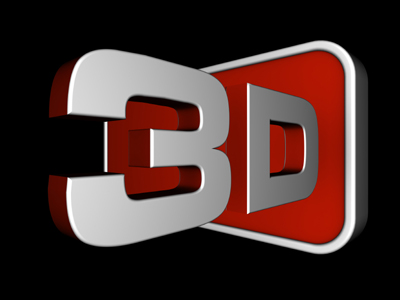Cubes, cylinders and spheres are all 3-D shapes.

# Shapes 2 (Very Easy)

Shapes are a very important part of maths and you will need to learn their names and their properties. Most shapes you come across are regular polygons, such as squares, hexagons and circles. But these are all 2-D shapes. You’ll also encounter 3 dimensional ones like cubes, cylinders and spheres.

This is the second of our Very Easy Eleven Plus maths quizzes on shapes. If you have already played the first then this one should be a doddle. Have a go and see if you can score ten out of ten.

Before you start, make sure that you read each question carefully and fully understand it before you pick your answer. Once you’ve mastered this, then move on to the next quiz. After all, the more practice you get with shapes, the better you will understand them and the more successful you will be in your exams. Good luck!

1.
How many corners and faces does a cube have?
8 corners and 6 faces
12 corners and 6 faces
6 corners and 8 faces
12 corners and 8 faces
Cubes are like 3-D squares. They have 8 corners (vertices), 6 faces (facets), and 12 edges
2.
Which 2-D shape has seven sides which are equal in length and joined at the same angle to each other?
A regular pentagon
A regular hexagon
A regular heptagon
A regular octagon
If the sides weren't all the same length, it would be called an 'irregular' heptagon. Polygons are closed shapes that have three or more straight sides: pentagon (5 sides), hexagon (6 sides), heptagon (7 sides), octagon (8 sides), nonagon (9 sides), decagon (10 sides). Try to remember these if you can
3.
The internal angles of a triangle always add up to how many degrees?
90o
180o
270o
360o
4.
How many sides does a quadrilateral have?
Three sides
Four sides
Six sides
Eight sides
Quadrilaterals have four sides (edges) and four corners (vertices). Squares and rectangles are both quadrilaterals
5.
Which of the following shapes is not a quadrilateral?
A rectangle
A square
A cube
A rhombus
Cubes are 3-D shapes. Quadrilaterals are 4-sided, 2-D shapes
6.
What is another term for a 3-D shape?
A solid
A polygon
A vertex
3-D shapes are also known as solids. Solids have volume whilst 2-D shapes are ‘flat’
7.
What do we call a 3-D shape with straight parallel sides and a circular top and bottom?
A sphere
A prism
A pyramid
A cylinder
Cylinders are everywhere: tin cans, rolling pins, pipes, candles, torches, toilet rolls, pencils, straws… the list goes on!
8.
What do we call a line which cuts a circle in half and passes through its centre?
Diameter
Circumference
Arc
The radius is half the diameter from the centre to the edge. The circumference is the distance around a circle, like the perimeter of other shapes. An arc is a part of the circumference
9.
What do we call a triangle with one right-angle corner?
A square triangle
A half-square triangle
A right-angled triangle
A perfect triangle
There are lots of different types of triangle besides right-angled: equilateral, isosceles, scalene, acute and obtuse. You don’t need to know about all of these yet – but you will do much better at secondary school if you learn them early
10.
A parallelogram whose sides are the same length is often called a ‘diamond’. What is another name for a ‘diamond’ shape?
Square
Trapezium
Kite
Rhombus
A rhombus is like a squashed square, and a parallelogram is like a squashed rectangle. Trapeziums have just one set of parallel sides
Author:  Frank Evans